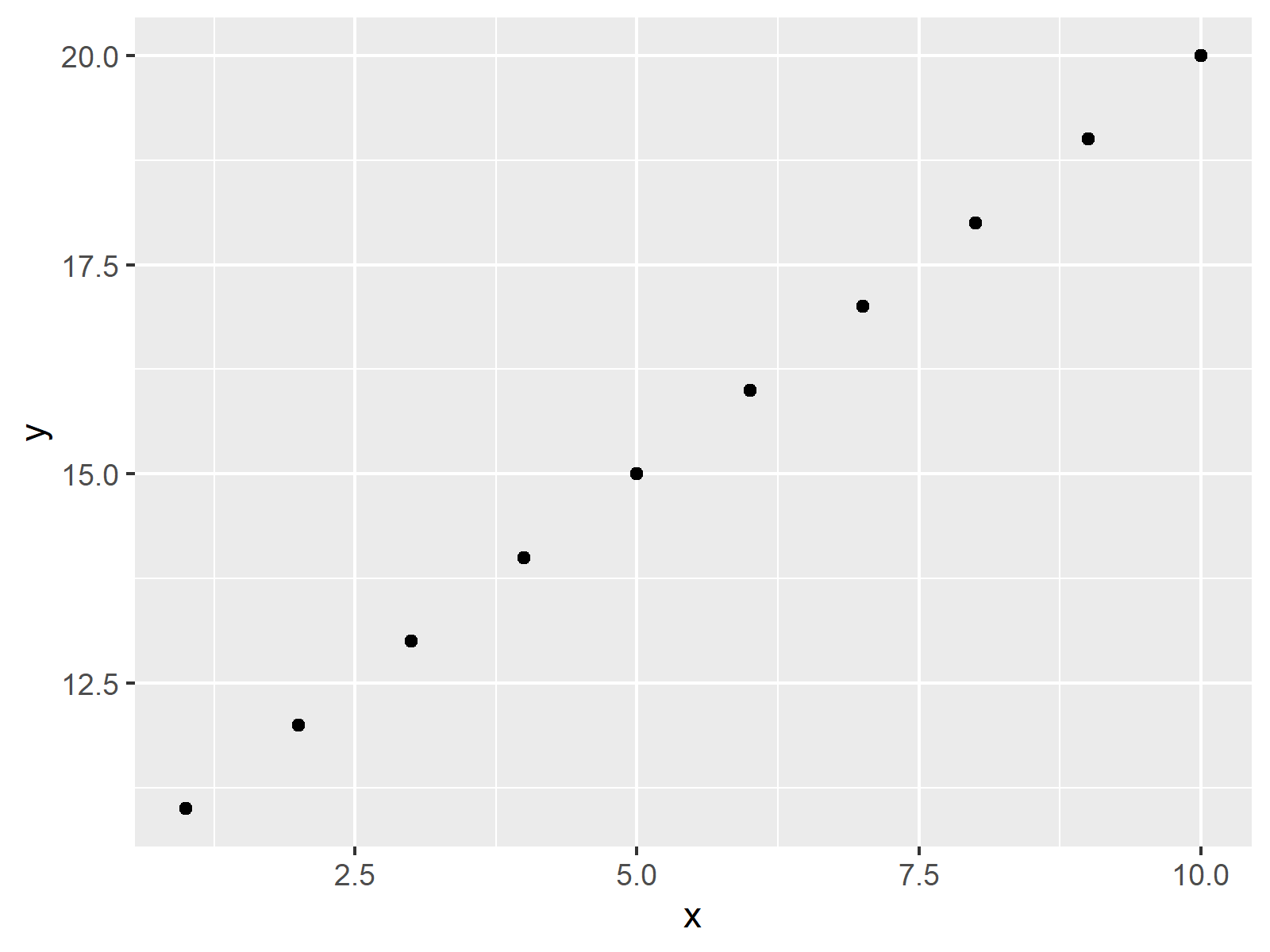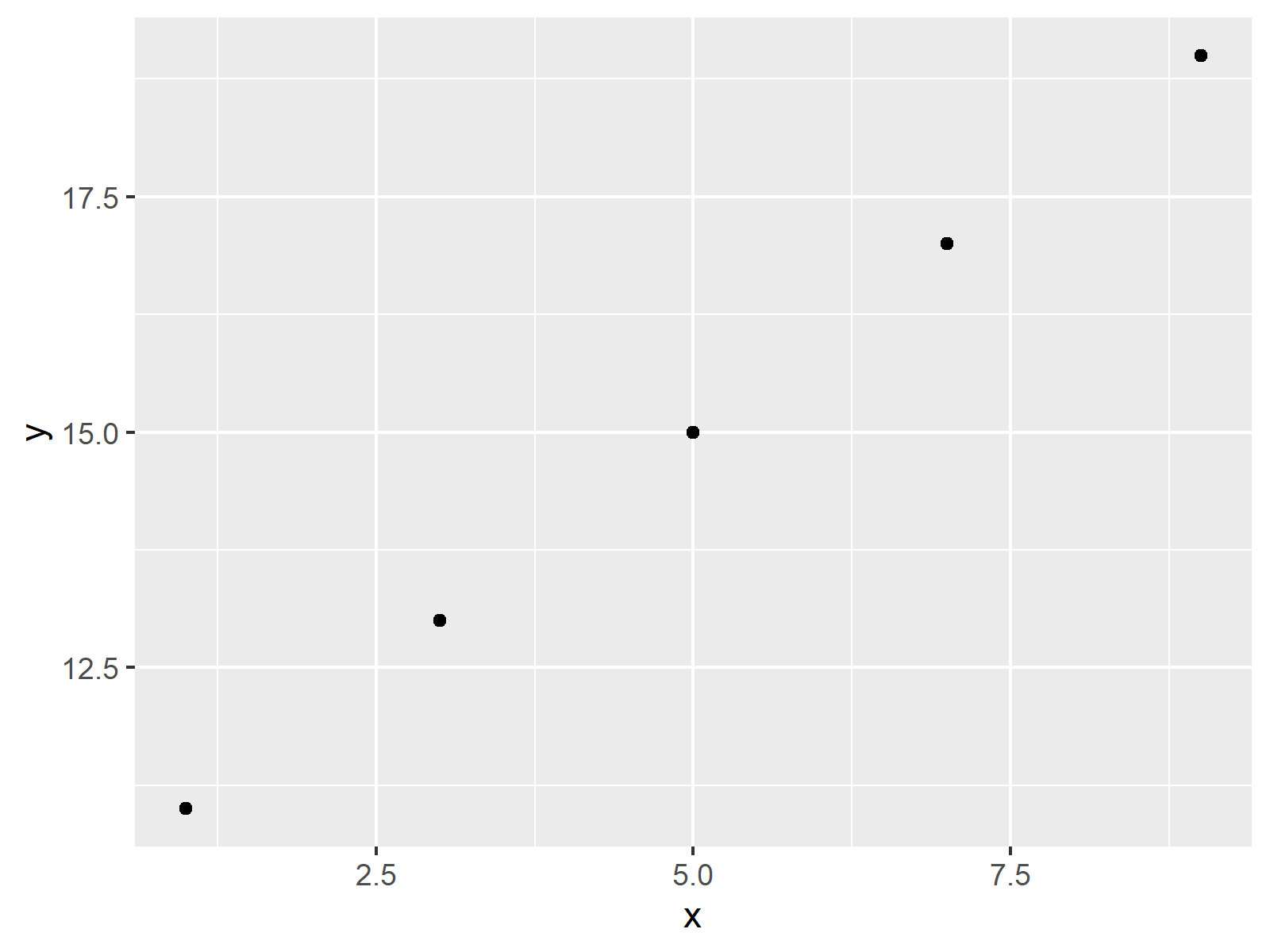# Draw ggplot2 Plot of Data Frame Subset in R (3 Examples)

In this tutorial you’ll learn how to create a ggplot2 plot of a data frame subset in R.

The content of the page is structured as follows:

Here’s the step-by-step process:

## Example Data, Packages & Default Graph

The following data is used as basement for this tutorial:

```data <- data.frame(x = 1:10,                       # Create example data
y = 11:20,
group = LETTERS[1:2])
data                                               # Print example data
#     x  y group
# 1   1 11     A
# 2   2 12     B
# 3   3 13     A
# 4   4 14     B
# 5   5 15     A
# 6   6 16     B
# 7   7 17     A
# 8   8 18     B
# 9   9 19     A
# 10 10 20     B```

The previous output of the RStudio console shows that the example data has ten rows and three columns. The variables x and y contain numeric values and the variable group contains a grouping indicator.

If we want to apply the functions of the ggplot2 package, we also have to install and load ggplot2:

```install.packages("ggplot2")                        # Install & load ggplot2
library("ggplot2")```

Now, we can plot our data as shown below:

```ggplot(data, aes(x, y)) +                          # Draw all data in ggplot2 plot
geom_point()```As shown in Figure 1, the previous R programming syntax created a ggplot2 scatterplot showing the data points of our entire data frame.

## Example 1: Creating ggplot2 Plot of Data Frame Subset Using Square Brackets

This example shows how to draw a data frame subset in a ggplot2 plot by using square brackets and the %in% operator to subset our data frame by logical condition in R.

Have a look at the following R syntax:

```ggplot(data[data\$group %in% "A", ], aes(x, y)) +   # Draw only subset of data
geom_point()```As shown in Figure 2, the previous R programming syntax created a ggplot2 plot that shows only one of the two groups contained in our data frame.

## Example 2: Creating ggplot2 Plot of Data Frame Subset Using subset() Function

Alternatively to square brackets (as shown in Example 1), we can also use the subset function before drawing our data:

```ggplot(subset(data, group %in% "A"), aes(x, y)) +  # Apply subset function
geom_point()```As shown in Figure 3, the previous R code plotted exactly the same ggplot2 graph as the R code of Example 1.

## Example 3: Create Data Frame Subset Before Plotting

Another alternative is that we create a data frame subset in the forefront of drawing our plot.

In step 1, we construct a new data frame…

`data_subset <- data[data\$group %in% "A", ]         # Extract certain rows of data frame`

…and in step 2, we create a plot based on the previously created data:

```ggplot(data_subset, aes(x, y)) +                   # Using reduced data frame in plot
geom_point()```As you can see in Figure 4, the previous syntax also created the same plot as in the previous examples.

## Video, Further Resources & Summary

Do you need more information on the content of this tutorial? Then you might watch the following video of my YouTube channel. In the video, I explain the R codes of this article in RStudio.

Please accept YouTube cookies to play this video. By accepting you will be accessing content from YouTube, a service provided by an external third party.If you accept this notice, your choice will be saved and the page will refresh.

In addition, you might want to read the related articles on my website. I have released several posts about similar topics such as variables, graphics in R, regression models, and dates.

This tutorial explained how to draw only selected rows of a data frame in a ggplot2 graphic in R programming. Don’t hesitate to let me know in the comments, in case you have further questions.

Subscribe to the Statistics Globe Newsletter